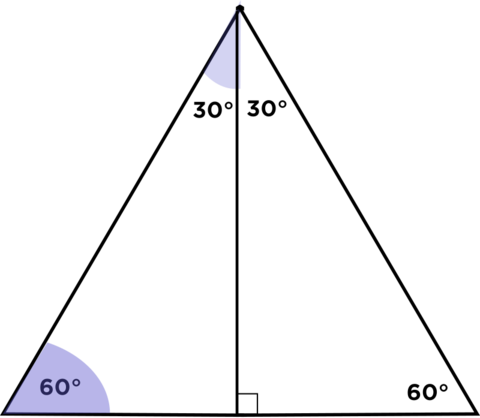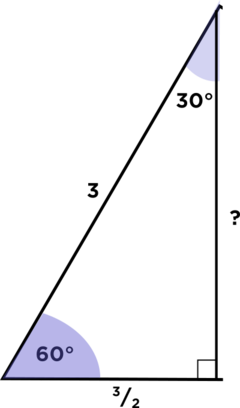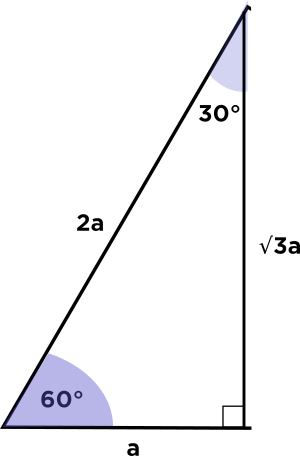# How did you know right away that it was 3/2 sqrt 3?

• Module 2 Week 1 Day 1 Your Turn Explanation

How did you know right away that it was 3/2 sqrt 3?

• Hi TSS Graviser!

The long leg of the 30°-60°-90° triangle from the lesson was $$\frac{3}{2} \sqrt{3}$$, and it might seem like this was done really fast, but that's because after seeing this special triangle many, many times in many different situations, it becomes almost second nature to people to be able to figure out the lengths of its sides. Don't worry, it will become second nature to you, too, after a long while!

The reasoning behind why this is true is very interesting and not that difficult to see. The first idea is that the $$30^{\circ} - 60^{\circ} - 90^{\circ}$$ right triangle is really just an equilateral triangle (a triangle with three equals sides) cut in half:

The sides of the equilateral triangle were all 3:So, after cutting the equilateral triangle in half, the short leg of our right triangle will be $$\frac{3}{2}$$:Now we have a right triangle, and we know two of the side lengths of it, so we know we can use the Pythagorean Theorem in order to find the other length.\begin{aligned} \left(\text{hypotenuse}\right) ^2 &= \left( \text{short leg}\right)^2 + \left(\text{long leg}\right)^2 \\ \left( \text{long leg} \right)^2 &= \left( \text{hypotenuse} \right)^2 - \left( \text{short leg} \right)^2 \\ \left( \text{long leg} \right)^2 &= 3^2 - \left( \frac{3}{2} \right)^2 \\ \left( \text{long leg} \right)^2 &= 9 - \frac{9}{4} \\ \left( \text{long leg} \right)^2 &= \frac{27}{4} \\ \left( \text{long leg} \right)^2 &= \frac{9}{4} \times 3 \\ \text{long leg } &= \sqrt{ \frac{9}{4} \times 3} \\ &= \frac{3}{2} \times \sqrt{3} \end{aligned}

After seeing a lot of $$30^{\circ}-60^{\circ}-90^{\circ}$$ triangles, you might start to notice a neat pattern:

• The hypotenuse is twice as long as the short leg

• The long leg is $$\sqrt{3}$$ times the short legWe can also see this by using the Pythagorean Theorem on the side lengths of this general triangle!

$$(2a)^2 = a^2 + (\sqrt{3}a)^2$$
$$4a^2 = a^2 + 3a^2$$

I hope this helps! Please don't hesitate to ask if you have any more questions!

Happy LearningThe Daily Challenge Team